Courses

# Chemistry Mock Test

## 45 Questions MCQ Test NEET Mock Test Series | Chemistry Mock Test

Description
This mock test of Chemistry Mock Test for NEET helps you for every NEET entrance exam. This contains 45 Multiple Choice Questions for NEET Chemistry Mock Test (mcq) to study with solutions a complete question bank. The solved questions answers in this Chemistry Mock Test quiz give you a good mix of easy questions and tough questions. NEET students definitely take this Chemistry Mock Test exercise for a better result in the exam. You can find other Chemistry Mock Test extra questions, long questions & short questions for NEET on EduRev as well by searching above.
QUESTION: 1

Solution:
QUESTION: 2

Solution:
QUESTION: 3

### The strongest acid among the following aromatic compounds is

Solution:

The strongest acid among the following aromatic compound is p-Nitrophenol. When strong electron withdrawing nitro group is present in para position, the loss of proton from -OH group becomes easy as the phenoxide ion is resonance stablizied and nitro group decreases electron density from phenoxide ion. This increases the acidic behaviour.QUESTION: 4

B(mix)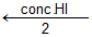(CH3)2C—O—CH3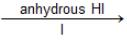A(mix)

Solution:
QUESTION: 5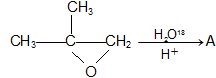, A is

Solution:
QUESTION: 6

50 ml of 1 M oxalic acid is shaken with 0.5 g wood charcoal. The final concentration of the solution after adsorption is 0.5 M. What is the amount of oxalic acid absorbed per gm of carbon?

Solution:
QUESTION: 7

Ferric chloride is applied to stop bleeding because

Solution:
QUESTION: 8

If same amount of electricity is passed through aqueous solution of AgNO3 and CuSO4 and the number of Ag and Cu atoms deposited are x and y respectively. Then

Solution:
QUESTION: 9

The e.m.f of the following Daniell cell at 298 k is EZn |ZnSO4(0.01M)| CuSO4(1.0M)| Cu When the concentration of ZnSO4 is 1.0 M and that of CuSO4 is 0.01 M, the e.m.f changed to E2. what is the relationship between E1 and E?

Solution:
QUESTION: 10

In the metallurgy of iron, when limestone is added to the blast furnace, the calcium ion ends up in

Solution:
QUESTION: 11

In acidic medium, KMnO4 oxidises FeSO4 solution. Which of the following statement is correct?

Solution:
QUESTION: 12

The decomposition of a substance follows first order kinetics. Its concentration is reduced to 1/8th of its initial value in 24 minutes. The rate constant of the decomposition process is

Solution:
QUESTION: 13

For the reaction system 2NO(g) + O2 (g) → 2NO2 (g) the volume is suddenly reduced to half its value by increasing the pressure on it. If the reaction is of first order with respect to O2 and second order with respect to NO, the rate of reaction will be

Solution:
QUESTION: 14

The freezing point of 0.1 M solution of glucose is -1.860C. If an equal volume of 0.3 M glucose solution is added, the freezing point of the mixture will be

Solution:
QUESTION: 15

The vapour pressure of benzene at a certain temp. is 640 mm Hg. A non-volatile-non-electrolyte and weighing 2.175g is added to 39.0g of benzene. The vapour pressure of the solution is 600 mm Hg. What is the molecular weight of the solid substance ?

Solution:
QUESTION: 16

In the laboratory, sodium chloride is made by burning sodium in the atmosphere of chlorine. The salt obtained is yellow in colour. The cause of yellow colour is

Solution:
QUESTION: 17

In a solid AB having the NaCl structure, ‘A’ atoms occupy the corners of the cubic unit cell. If all the face-centred atoms along one of the axes are removed, then the resultant stoichiometry of the solid is

Solution:
QUESTION: 18

The products A and B in the following sequence of reactions

R1 — CH2NO2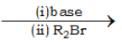A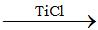B

Respectively are:

Solution:
QUESTION: 19

An organic compound ‘A’ having molecular formula C2H3N on reduction gave another compound ‘B’. Upon treatment with nitrous acid, ‘B’ gave ethyl alcohol. On warming with chloroform and alcoholic KOH, it formed an offensive smelling compound ‘C’. The compound ‘C’ is

Solution:
QUESTION: 20

The missing product x in the following reaction will be

3SiF4 + 4H2O → Si(OH)4 + x

Solution:
QUESTION: 21

A radioactive sample has half life of 1500 years. A sealed tube containing 1 g of a sample will contain g of the sample after 3000 years.The missing figure is

Solution:
QUESTION: 22

The basis for carbon – 14 dating method is that the

Solution:
QUESTION: 23

An aqueous solution of sodium sulphate is electrolysed using inert electrodes. The products at the cathode and anode are respectively

Solution:
QUESTION: 24

Which of the following statements is false for alkali metals ?

Solution:
QUESTION: 25

Which of the following volume (V)-  temperature (T) plots represents the behaviour of one mole of an ideal gas at one atmospheric pressure?

Solution:
QUESTION: 26

Two glass bulbs A and B are connected by very small tube having a stop cock. Bulb A has a volume of 100 ml and contained the gas while bulb B was empty and had a volume of 150 ml. On opening the stop-cock, the pressure of the gas in bulb A will fall down to

Solution:
QUESTION: 27

Equal volumes of two gases which do not react together are enclosed in separate vessels. Their pressures at 100 mm and 400 mm respectively. If the two vessels are joined together, then what will be the pressure of the resulting mixture (temperature remaining constant)?

Solution:
QUESTION: 28

If the nitrogen atom had electronic configuration 1s7, it would have energy lower than that of the normal ground state configuration1s2 2s2 2p3 because the electrons would be closer to the nucleus. Yet 1s is not observed. It violates

Solution:
QUESTION: 29

What transition in the hydrogen spectrum would have the same wavelength as the Balmer transition, n = 4 to n = 2 in the He+ spectrum?

Solution:
QUESTION: 30

An element (X) forms compounds of the formula XCl3,X2O5 and Ca3X2 but does not form XCl5. Which of the following is the element X ?

Solution:
QUESTION: 31

H2O has a net dipole moment while BeF2 has zero dipole moment because

Solution:
QUESTION: 32

For a reaction R1,(Δ) G = x kj mol-1. For a reaction R2, (Δ)G = y kj mol-1 Reaction R1 is non-spontaneous but along with R2 it is spontaneous. This means that

Solution:
QUESTION: 33

(Δ)G for the reaction Ag2O → 2 Ag + 1/2 O2 at a certain temperature is found to be – 10.0 kj mol-1. Which one of the following statements is correct at this tempertature?

Solution:
QUESTION: 34

A solution of 500 ml. of 0.2 M KOH and 500 ml of 0.2 M HCl is mixed and stirred; the rise in temperature is T1. The experiment is repeated using 250 ml each of solution, the temperature rise is T2. Which of the following is true?

Solution:
QUESTION: 35

Which of the following oxides of nitrogen will be the most stable one?

Solution:
QUESTION: 36

An organic compound, C3H6O does no give a precipitate with 2,4-dinitrophenylhydrazine reagent and does not react with metallic sodium. It could be

Solution:
QUESTION: 37

In which of the following solvents will AgBr have highest solubility ?

Solution:
QUESTION: 38

For the gaseous reaction N2 + 3H2 → 2NH3 the partial pressure of  H2 and N2 are 0.4 and 0.8 atm respectively. The total pressure of the entire system is 2.8 atm. What will be the value of K, if all concentrations are given in atmospheres?

Solution:
QUESTION: 39

If the solubility of lithium sodium hexafluoroaluminate, Li3Na3 (AlF6)2 is ‘a’ mole/litre,the solubility product is equal to

Solution:
QUESTION: 40

If S1, S2, S3 and S4 are the solubilities of AgCl in water, in 0.01 M CaCl2 in 0.01 M NaCl and in 0.05 MAgNO3 respectively at a certain temperature, the correct order of solubilites is

Solution:

The concentration of common ion ∝ 1/solubility

The order of solubility of AgCl  : S1 > S3 > S2 > S4

QUESTION: 41

A mole of N2H4 loses ten moles of electrons to form a new compound Y. Assuming that all the nitrogen appears in the new compound, what is the oxidation state of nitrogen in Y? (there is no change in the oxidation number of hydrogen)

Solution:
QUESTION: 42

An organic compound X on treatment with acidified k2Cr2O7 gives compound Y which reacts with I2 and sodium carbonate to form triiodomethane. The compound X can be

Solution:
QUESTION: 43

A compound A has molecular formula C2Cl3OH. It reduces Fehling’s solution and on oxidation gives a monocarboxylic acid B. A is obtained by the action of Cl2 on ethyl alcohol.A is

Solution:
QUESTION: 44

The end product ‘Z’ in the following reaction,  Ethylamine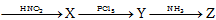is

Solution:
QUESTION: 45

Replacement of Cl of chlorobenzene to give phenol requires drastic conditions but chlorine of 2, 4-dinitrochlorobenzene is readily replaced.This is because

Solution: